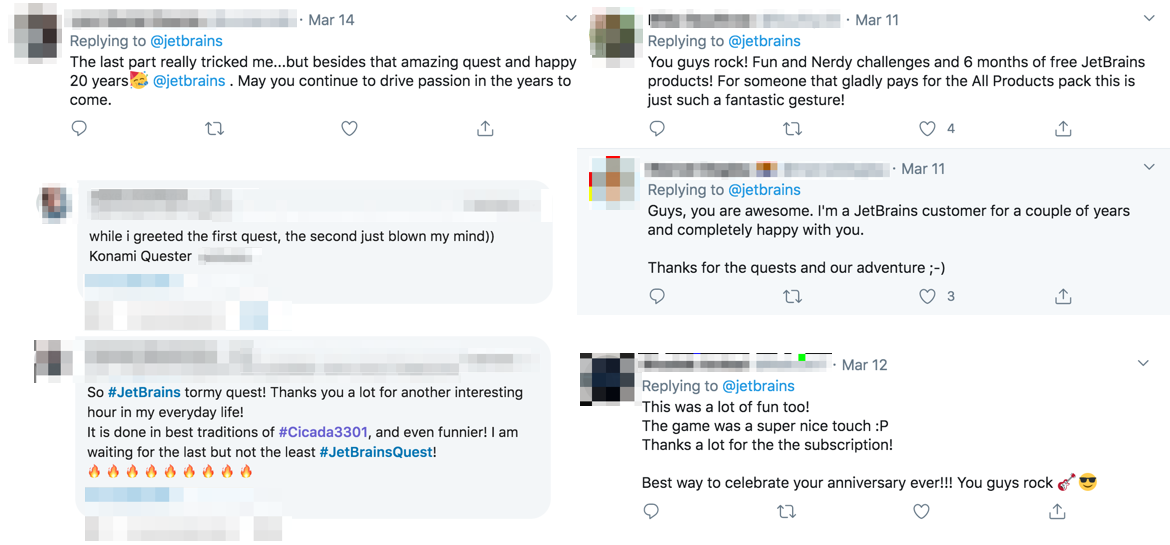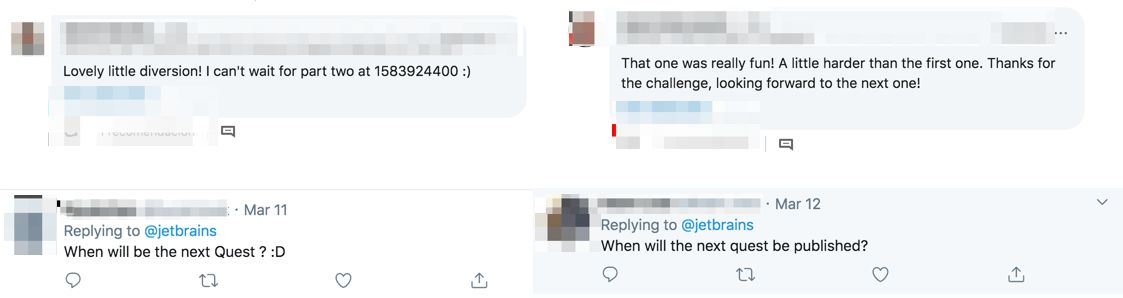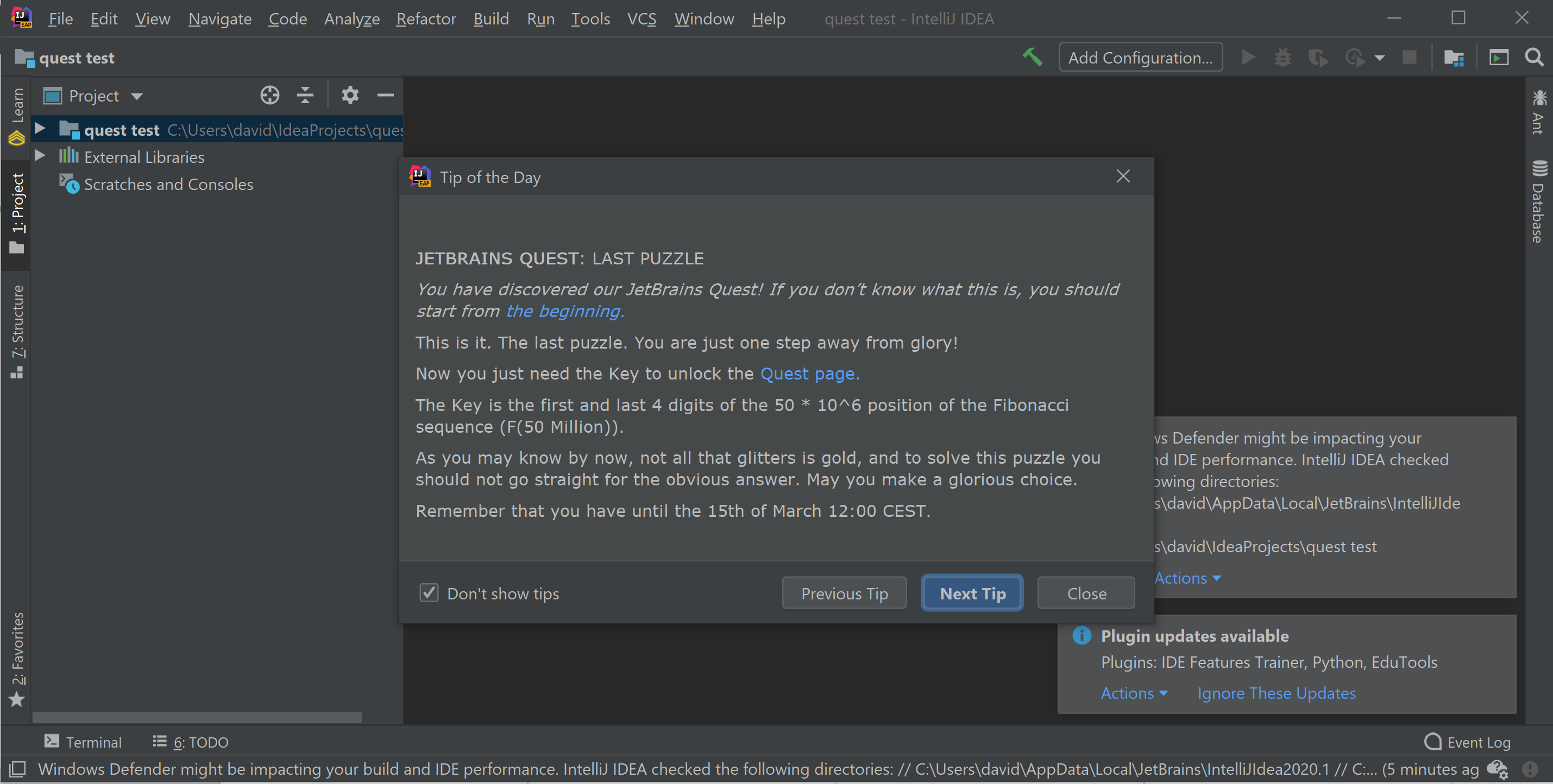JetBrains

# JetBrains Quest 到底是什么？

JetBrains 有很多开发人员，其中很多人都喜欢玩游戏 – 具有挑战性的游戏。

我们提出了创建寻宝游戏的想法：每个谜题的解决方案都是下一个谜题的提示。我们将所有的谜题彩蛋隐藏于 JetBrains 的各种地方。

JetBrains Quest 是一系列谜题，遍布所有不同的 JetBrains 页面和产品。游戏包含 3 个任务，每个任务有 4 – 6 个谜题可解决。第一个任务相对容易，使人们有机会弄清楚要期望什么。随着前进，难度也增加了。import math def last_fib_digits(fib_number, last_digits): prev, cur = 0, 1 q = 10 ** last_digits while fib_number > 0: prev, cur = cur, prev + cur fib_number -= 1 cur %= q return prev def first_fib_digits(fib_number): phi = (math.pow(5.0, 0.5) + 1) / 2 logF = fib_number * math.log10(phi) – 0.5 * math.log10(5.0) return math.pow(10.0, logF – int(logF)) print(last_fib_digits(50000000, 4)) print(first_fib_digits(50000000))

– JetBrains Quest 团队